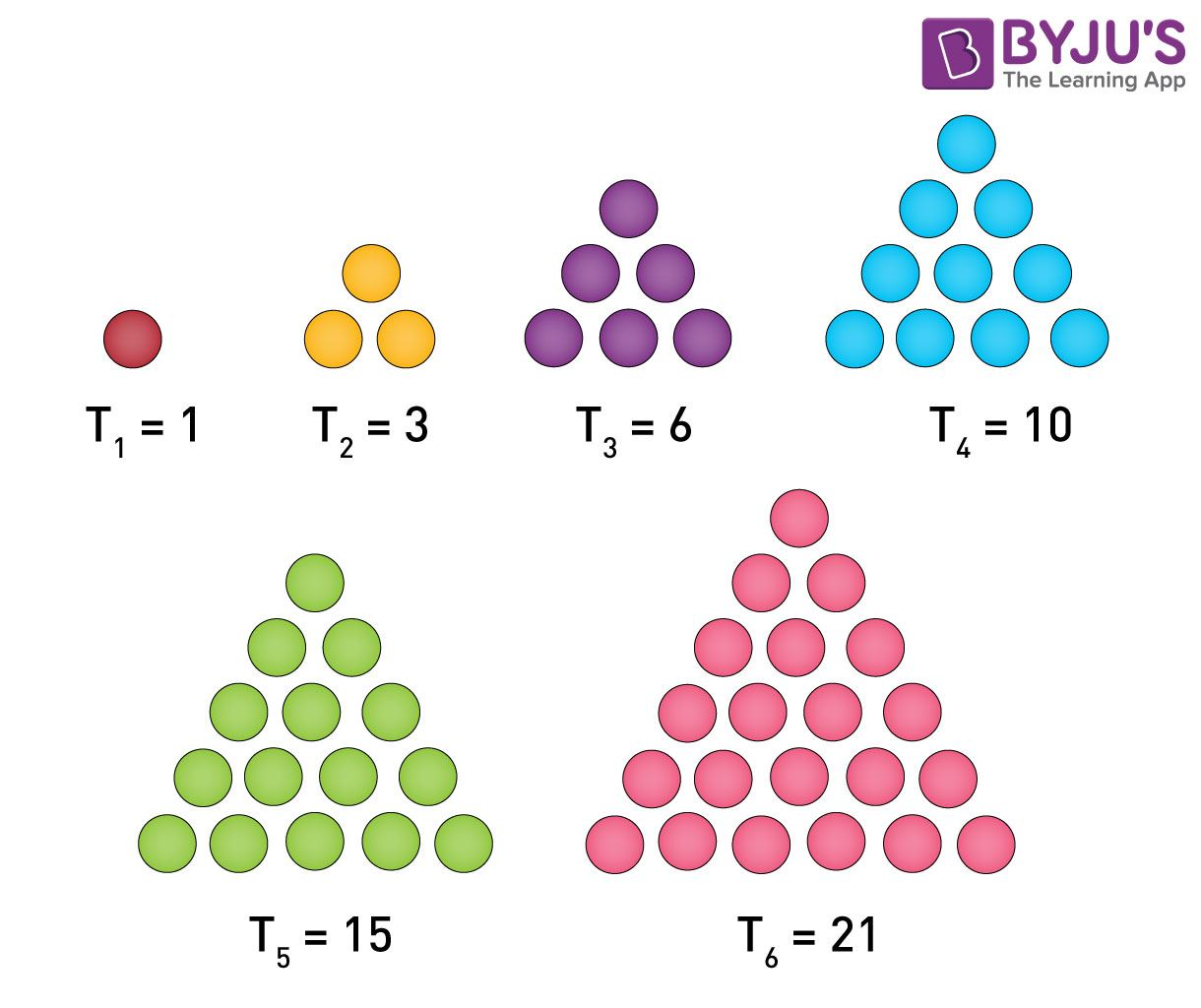# Triangular Numbers Sequence

The triangular number sequence is the representation of the numbers in the form of equilateral triangle arranged in a series or sequence. These numbers are in a sequence of 1, 3, 6, 10, 15, 21, 28, 36, 45, and so on. The numbers in the triangular pattern are represented by dots. The sum of the previous number and the order of succeeding number results in the sequence of triangular numbers. We will learn more here in this article.

## What is Triangular Number?

A triangular number Tn is a figurative number that can be represented in the form of an equilateral triangular grid of elements such that every subsequent row contains an element more than the previous one.### List Of Triangular Numbers

0, 1, 3, 6, 10, 15, 21, 28, 36, 45, 55, 66, 78, 91, 105, 120,136, 153, 171, 190, 210, 231, 253, 276, 300, 325, 351, 378, 406, 435, 465, 496, 528, 561, 595, 630, 666, 703, 741, 780, 820, 861, 903, 946, 990, 1035, 1081, 1128, 1176, 1225, 1275, 1326, 1378, 1431, and so on.

## Sum of Triangular Numbers

In the pattern of triangular numbers you will see, the next number in the sequence is added with an extra row. Let us explain in detail.

• First number is 1
• In number 2,  a row is added with two dots to the first number
• In number 3, a row is added with three dots to the second number
• Again, in number 4, a row is added with four dots to the third number and so on

So the sequence formed here is in the pattern:

1, 1 + 2, 1 + 2 + 3, 1 + 2 + 3 + 4,  and so on.

## Formula For Triangular Number Sequence

Triangular numbers correspond to the first-degree case of Faulhaber’s formula.

$$\begin{array}{l}T_{n}=\sum_{k=1}^{n}k=1+2+3+…+n=\frac{n(n+1)}{2}\end{array}$$

Where,

(n+1)/2 is the binomial coefficient. It represents the number of distinct pairs that can be selected from N+1 objects.

Further, (n+1)/2 can be expressed as:

$$\begin{array}{l}\frac{(n+1)!}{(n+1-2)!2!}\end{array}$$

This can be further simplified as [n(n+1)]/2.

By the above formula, we can say that the sum of n natural numbers results in a triangular number, or we can also say that continued summation of natural numbers results in a triangular number. The sum of two consecutive natural numbers always results in a square number.

$$\begin{array}{l}T1+T2= 1+3=4=2^{2}\end{array}$$
and
$$\begin{array}{l}T2+T3= 3+6=9=3^{2}\end{array}$$

All even perfect numbers are triangular numbers, and every alternate triangular number is a hexagonal number given by the formula:

$$\begin{array}{l}M_{P}2^{p-1}=\frac{M_{p}(M_{p}+1)}{2}=T_{Mp}\end{array}$$

Where MP is a Mersenne prime.

For example, the third triangular number is (3 × 2 =) 6, the seventh is (7 × 4 =) 28, the 31st is (31 × 16 =) 496, and the 127th is (127 × 64 =) 8128.

## Video LessonQuiz on Triangular Numbers